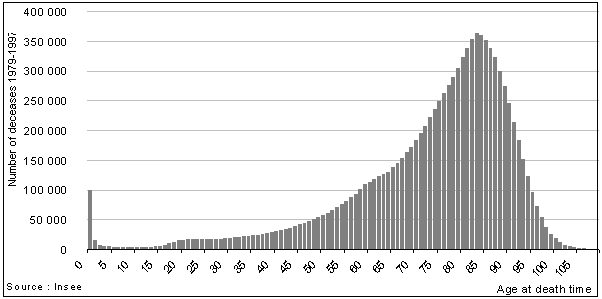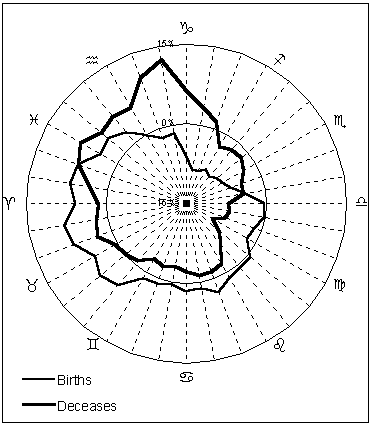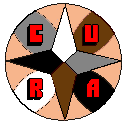The Manifesto | The Dominion | Texts and Articles | Review | Links | ACCUEIL (FR) | HOME (EN)

 A Link between Birth and Death by Didier Castille

Summary: Deceases registered in France between 1979 and 1997 have been examined as a whole, according to the zodiacal longitudes of the Sun at the birth time and at the death time. From this examination, an questionnable statistical association emerges. Deceases occuring around the birthday, more or less thirty days, might be more numerous than predicted by chance.

Hypothesis and method

 The hypothesis is that there is no statistical link between somebody's date of death and date of birth. In order to test this hypothesis, the study is based on the French registry offices files that are produced by Insee (National Institut of Statistics and Economic Studies). These yearly files provide, for each deceased person, the date of death and the date of birth. The preliminay work consisted in associating the population data with an astrological calendar to assign a solar longitude (at 12 noon G.M.T.) to each date. According to these longitudes, twelve thirty-degrees wide classes were built. With this operation, 216 observations were identified that contain at least one wrong date (February 30th or April 31st, for example). Method: the zodiac The astrological zodiac is a reference system that locates the Sun, the Moon and the solar system planets in space relative to Earth. The point of origin of this reference system is called the "vernal point", meaning the Spring equinoctial point. The zodiac is a time measuring tool : a date of birth or a date of death may be translated by the longitude of the Sun on the ecliptic. For example, some people may be born at Aries (Sun's longitude betwen 0° and 30° = Aries) and may die at Leo (Sun's longitude betwen 120° and 150° = Leo). Here, the astrological sign where the Sun was at 12 noon G.M.T. was assigned to each date of birth and to each date of death. In this, it was taken into account that the entry of the Sun in a given sign does not occur at fixed dates.

In a first step, a Chi-square independence test was performed. The deceases were broken down according to the birth time astrological sign and to the decease time astrological sign.

Next, a theoretical table was calculated. For each of the 144 cells, the total number of the column to which the cell belongs was multiplied by the total number of the row to which the cell belongs. The result was divided by the total number of the table.

This procedure neutralized the birth and death distribution disparities that naturally exist between signs (cf. appendix). Then, for each cell, the deviation of the cell frequency from the theoretical value was calculated, squared and divided by the theoretical value. This calculation shows, for each cell, the amplitude of the shift compared to the theoretical value. Next, the Chi-square value was calculated by totalizing the 144 relative deviations. Each relative deviation is called "contribution to the Chi-square".

The global significance of the deviation is deducted from both Chi-square value and number of cells. It is estimated from the risk taken by rejecting the null hypothesis, which means there is no link between date of birth and date of death.

After that, each contribution to the Chi-square was analysed individually in order to estimate the influence of each combination of signs to the global deviation.

In a second step, for each decease, the angle formed by the longitude of the Sun at birth time and the longitude of the Sun at death time was calculated. In others words, it corresponds to the number of days separating the day of decease from the nearest birthday. According to the value of the angle, the population was distributed into thirty-six classes of ten degrees each. Next the distribution was compared to a theoretical uniform distribution.

The French mortality statistics

In collaboration with the registry offices of the town councils, Insee keeps current yearly computer files for demographical or sociological studies. Since 1979, the files mention the complete date of birth and date of decease. The files which were used for this study cover the years 1979 to 1997 and represent a total of 10 192 111 deceases.

Deceases of children under one are numerous. They represent 1% of the whole population when, for example, deceases of one year old children represent only 0.15%. For this special case, it is clear that the day of birth is close to the day of decease. To avoid their mechanical influence on the test, the deceases of children under one were excluded from the study field. Then, the study population contained 10 081 752 deceases.

First results

The independence test produced a Chi-square value of 229.69. With such a value, the risk taken by rejecting the null hypothesis (meaning there is no association between solar signs at birth and at death) is very slight.

The rows of the following table correspond to the solar sign at birth, the column correspond to the solar sign at decease. The cell that represents the cross between a row and a column presents the deviation of the cell from the expected value. For example, the number of persons who were born as the Sun was transiting Aries and who died as the Sun was transiting Taurus is greater than theoretical (+109 persons). In the same way, the number of persons who were born as the Sun was transiting Aries and who died as the Sun was transiting Virgo is less than theoretical (-163 persons).

Distribution of deceases according to the solar sign at birth and to the solar sign at death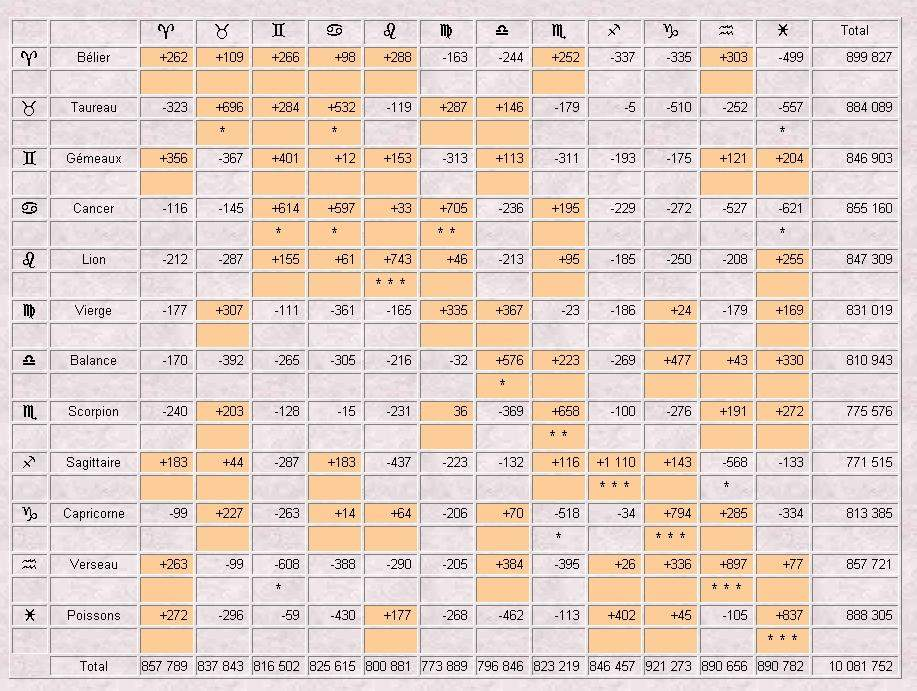The colored cells in this table highlight those combinations for which the actual number of deceases is greater than the theoretical number. The amplitude indicators of the contribution (the asterisks beneath a value) can be translated as follows:

 The value of the contribution is: the indicator is: It means that the deviation is: superior to 7,88 * * * significant with a risk of 0.5% at the most comprised between 6.63 and 7.88 * * significant with a risk of 1% at the most comprised between 3.84 and 6.63 * significant with a risk of 5% at the most inferior to 3.84 not significant

By more carefully examining the deceases distribution table and, especially the contributions to the Chi-square, another phenomenon appears. There is a diagonal series of actual frequencies that exceed theoretical frequencies for persons born and deceased under the same solar sign. The table presenting the deviation of the actual frequencies from the expected values shows a structure.

The total of the Chi-square contributions of the 12 combinations which form the diagonal of excess observations is quite high. It reaches 83.9 or 36.5% of the Chi-square value. Theoretically, it should be 19.1 (a twelfth of the Chi-square value). This diagonal set of frequencies is very particular and can not be attributed to chance.

The number of deceases generating this diagonal exceeds by 1% the theoretical number (+7 906 deceased persons).

Controls

In order to verify the previous results, another Chi-square independence test was performed on a fictitious population.

This fictitious population was built by dividing the original file in two parts, one containing the birth dates and the other containing the dates of decease, and by creating random combinations between the two groups.

The conclusion of the control test is that the null hypothesis can be rejected with an rerror risk of 5%. In other words, the test leads to the same conclusions, whether it was performed on a real population or on a fictitious population. Consequently, the date of decease might not be linked to the date of birth.

Nevertheless, the significance levels of both of the tests are quite different (229.69 for the real population, 147.68 for the fictitious population). More, the diadonal of excess observations that was observed in the real population does not exist any more in the fictitious distribution.

Solar longitudes proximity

Here the study concentrates on the synastric aspect formed by the Sun's longitude at the date of birth and the Sun's longitude at the date of decease. The aspects were calculated for each deceased person and segmented into thirty-six classes of ten degrees each. Next, the distribution was compared to a theoretical uniform distribution and a one-way Chi-square test was performed in order to measure the global amplitude of the deviations.

The Chi-square value reaches 226.80. This value permits rejection of the null hypothesis (meaning that decease date is independent from birth date) with a very small risk of error.

11 classes out of 36 are characterized by an above theoretical frequency. Among these 11 classes, 7 are adjoining. These 7 classes comprise a total of 1 972 097 deceased persons, representing a surplus of 11 756 deceases compared to the theoretical value (+0.6%).

Distribution of deceases according to the angle formed
by the Sun's longitude at birth and the Sun's longitude at death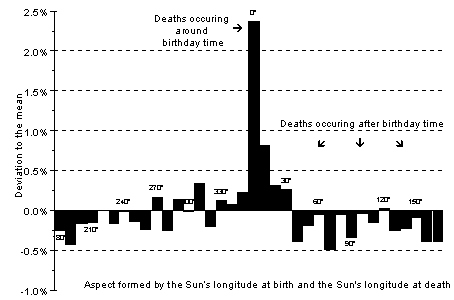Conclusion

The study of 10 081 752 deceases that occured between 1979 and 1997 and which were registered by the French registry offices does not permit to indisputably reject the hypothesis meaning that date of birth and date of decease are statistically independent.

A first approach shows that this hypothesis can be rejected. But, generating suspicions, a test performed on a fictitious population permits to reject the hypothesis as well. Nevertheless, the examination of the contributions to the Chi-square unexpectedly reveals that the distribution is structured, what is contrary to the chance principle.

On the other hand, a second approach shows that the null hypothesis can by undeniably rejected. People who die around their birthday, more or less thirty days, are more numerous than in theory.

For all that, shall we see here a mystery? The research should be extended by studying the reasons why the decease occured. Maybe suicides are more frequent around birthdays. We can also think about these birthday parties that tragically end, for example, because of a car crash or a too violent emotion. As many factors which, however, come under the astrologers' cyclic conception of life.

Appendix